#Method of Undetermined Coefficient or Guessing MethodThis method is based on a guessing technique. That is, we will guess the form of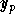and then plug it in the equation to find it. However, it works only under the following two conditions:

Condition 1: the associated homogeneous equations has constant coefficients;
Condition 2: the nonhomogeneous term g(x) is a special form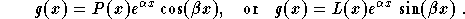where P(x) and L(x) are polynomial functions.
Note that we may assume that g(x) is a sum of such functions (see the remark below for more on this).

Assume that the two conditions are satisfied. Consider the equation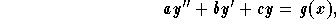where a, b and c are constants and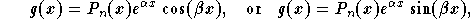where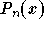is a polynomial function with degree n. Then a particular solutionis given by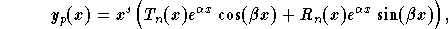where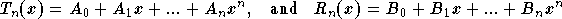,

where the constants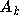and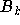have to be determined. The power s is equal to 0 if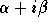is not a root of the characteristic equation. Ifis a simple root, then s=1 and s=2 if it is a double root.

Remark: If the nonhomogeneous term g(x) satisfies the following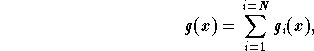where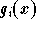are of the forms cited above, then we split the original equation into N equations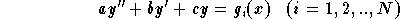then find a particular solution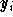. A particular solution to the original equation is given by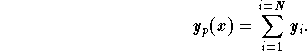Summary:Let us summarize the steps to follow in applying this method:

(1)
First, check that the two conditions are satisfied;
(2)
If the equation is given as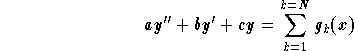,

where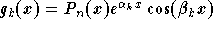or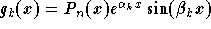, where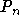is a polynomial function with degree n, then split this equation into N equations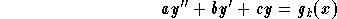;

(3)
Write down the characteristic equation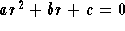, and find its roots;
(4)
Write down the number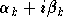. Compare this number to the roots of the characteristic equation found in previous step.
(4.1)
Ifis not one of the roots, then set s = 0;
(4.2)
Ifis one of the two distinct roots, set s = 1;
(4.3)
Ifis equal to both root (which means that the characteristic equation has a double root), set s=2.
In other words, s measures how many timesis a root of the characteristic equation;
(5)
Write down the form of the particular solution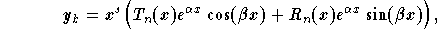where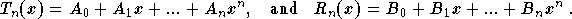(6)
Find the constants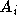and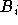by plugginginto the equation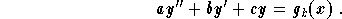(7)
Once all the particular solutionsare found, then the particular solution of the original equation is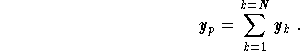[Differential Equations] [First Order D.E.] [Second Order D.E.]
[Geometry] [Algebra] [Trigonometry ]
[Calculus] [Complex Variables] [Matrix Algebra]S.O.S MATHematics home page

Do you need more help? Please post your question on our S.O.S. Mathematics CyberBoard.Author: Mohamed Amine Khamsi